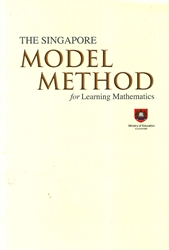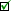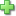# Singapore Model Method for Learning Mathematics

Price: \$32.00
Used Price: \$22.00 (1 in stock)Condition Policy

See series description for full review.

The Singapore Model Method is a pedagogical strategy that was developed by a team of curriculum specialists in the Singapore Ministry of Education in the early 1980’s to address the issue of students having difficulty with word problems in early years of school. It has since become a distinguishing feature of the Singapore primary mathematics curriculum.

Using this method, students represent the information in the problem pictorially using bars to represent numbers. The model show explicitly the problem structure, the known and unknown quantities, and provides a visual tool that enables students to determine what operations to use to solve the word problems.

This monograph goes through the various types of models, primarily using examples from the second edition of Primary Mathematics. These types are the part-whole and comparison model for addition and subtraction, the part-whole and comparison models for multiplication and division, the part-whole and comparison model for fractions, the part-whole and comparison model for percentage, the comparison model for ratios, and the change model. It then goes on to discuss applying a unitary approach to solving the problems, using the information from the model, and gives examples of how the Model Method can be used in problem solving, using more challenging problems. It shows how the Model Method facilitates Polya’s four-step problem solving processes and explains how students use a synthetic approach followed by an analytic approach when using the Model Method. Finally, it shows how the Model Method can be integrated with the algebraic method to help students develop competence and confidence in using the algebraic method by providing examples and discussing various methods of solutions, relating the Model Method to algebraic solutions. Included are references to numerous articles on the model-drawing method, mathematical problem solving, and mathematics education in Singapore.

This book is a valuable resource for anyone wanting a general overview of the Model Method that includes examples covering all levels of Primary Mathematics for grades 3 - 6, where it is primarily used, as well as ideas for how to use the Model Method to help students visualize and conceptualize a problem so that they can formulate an algebraic expression to solve it.

This book describes the Model Method and has many examples, but it does not include lessons for teaching students the method at various grade levels. It is about Model Drawing as taught in Singapore, and does not supply any examples of any U.S. adaptations of the Model Method, such as an 8-Step Model Drawing method. Such adaptations are not used in Singapore, are more limited in scope, and are adaptations meant for the relatively simpler problems in U.S mathematics textbooks.

The monograph is organized into seven chapters.

• Chapter 1 provides an overview of the Mathematics Framework and the Model Method as key features of the Singapore mathematics curriculum.
• Chapter 2 highlights the evolution of the Mathematics Framework over the last two decades
• Chapter 3 and 4 illustrate the use of pictorial models in the development of the concepts of the four operations as well as fraction, ration and percentage.
• Chapter 5 explains and discusses how the Model Method is used or solving structurally complex word problems at the primary level.
• Chapter 6 illustrates how the Model Method can be integrated with the algebraic method to formulate algebraic equations for solving problems.
• Chapter 7 concludes the monograph by discussing some perspectives of problem solving that account for the success of the Model Method and the connection between the Model Method and the algebraic method.
Did you find this review helpful?
Related Categories
Recommended for...
Customer ReviewsClick here to write a review